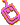Friday 4th December 2020# Classroom Key Stage 2 / KS2 - Maths Activities & Resources

## Welcome to the Maths section of the Key Stage 2 classroom. Within KS2 Maths you will find online @school assessment tests that will help children to enhance their learning and revise the subject of Maths at Key Stage 2 level.

•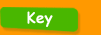•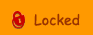•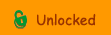••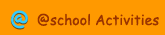•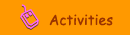•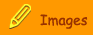###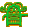Area

Area - Find the area of each grid in this interactive activity.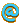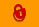Area  Year 6 - Interactive activity to calculate the area of compound shapesArea of Cubes - Interactive activity to calculate the surface area of a cube.Area of Right-angled Triangles - Interactive activity to calculate the area of right-angled trianglesCalculate the Area of Rectangles - Interactive activity about a formula for calculating area.Calculate the Area of Rectangles 2 - Interactive activity about a formula for calculating area.Comparing Areas - Interactive area activity.Measuring Shapes - Calculate the perimeters of different shapes - from easy to hard.